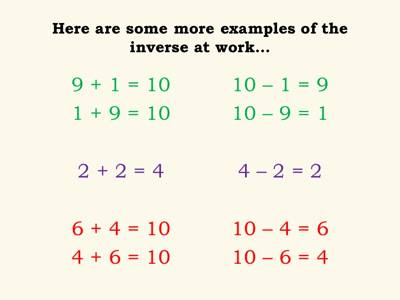Inverse relationship of Addition and Subtraction

Inverse relationship is a relationship between two numbers in which an increase in the value of one number results in a decrease in the value of the other number. The inverse relationship is also known as negative correlation in regression analysis; this means that when one variable increases, the other variable decreases, and vice versa.

There is an inverse relationship between addition and subtraction.

If a math fact is considered, for example 3 + 7 = 10. Then the following are also true:

• 10 – 3 = 7
• 10 – 7 = 3

Similar relationships exist for subtraction, for example 10 – 3 = 7. Then the following are also true:

• 3 + 7 = 10
• 7 + 3 = 10

The reason for this is that we are dealing with an equation. An equation is balanced or the same on either side of the equals (=) sign. If exactly the same thing is done to both sides of the equation, it will still be balanced or equal.

In the example above we start with the equation 3 + 7 = 10

• Subtract the same number from both sides 3 + 7 – 3 = 10 – 3
• On the left side the 3 and -3 produce 0 which leaves 7 = 10 – 3
• Turning the equation around to be in more normal form 10 – 3 = 7.Generally, subtraction facts are harder for children to learn than addition facts. If a child knows that 6 + 9 = 15, and he or she sees the subtraction sentence 15 – 9 = __, the child can think, 9 and what are 15? This use of thinking of the related addition fact when children encounter a subtraction fact they don’t know should be encouraged. Children often find themselves either counting up or counting back to solve subtraction, and that is inefficient. If children learn the important inverse relationship between addition and subtraction, subtraction facts will become much easier. As you work with the children, use questions that encourage this strategy of the inverse relationship between addition and subtraction.

Information Source: